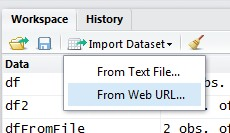# R - (Object|Variable|Symbol)

R provides a number of specialized objects. They are created (instantiated), used and referenced through variable (known as symbol).

• When you read the term object in the documentation, you can interchange it with the term variable (known as symbol)
• The variable (known as symbol) are themselves objects

The variable (symbol) references objects.

The symbols are themselves objects and has wide ranging effects.

R have some reserved variable (system variable)

## Example

• Numeric
``````> x=1
# Implicit printing of the variable
> x
 1
# Explicit printing of the variable
> print(x)```
```

The  indicates that x is of the vector class

``````> x+x
 2```
```

## Structure

### Type

The type determine the internal storage and can be seen as the primitive type of R.

### Class

The class properties permits R to have an object like behavior.

R supports several class (data structure) for an object:

And you can create yours.

### Attribute

All objects except the NULL object can have one or more attributes.

Common R objects attributes are:

• the element names,
• dim(ension) attribute (e.g. matrices, arrays)
• dimnames: the size and the name of each dimension of the object
• class which stores the name of the class of the object
• user-defined attributes

Attributes of an object can be listed using the attributes() function.

``````# data.frame object
x=data.frame(a=1:4,b=1:4,c=1:4)
attributes(x)```
```
``````\$names
 "a" "b" "c"

\$row.names
 1 2 3 4

\$class
 "data.frame"
```
```

The value of a specific attribute can be obtained using the attr() function (or NULL if the attribute is not defined).

For example,

``````# data.frame object
x=data.frame(a=1:4,b=1:4,c=1:4)
attr(x, "class")```
```
`````` "data.frame"
```
```

## Management

### Rename

``````newname <- oldname
rm(oldname)```
```

### List

List current objects (objects in the workspace)

````ls()`
```

ls shows you the variables

``````> x <- rnorm(50)
> y <- rnorm(x)
> ls()```
```
`````` "x" "y"
```
```

### Delete

``````rm(object)

# Delete all variables for the environment
rm(list = ls())```
```
``````> rm(x, y)
> ls()```
```
``````character(0)
```
```

### Copy

````newobject <- edit(object)`
```

### Serialization

apropos (or find) returns a character vector of all objects matching the text searched.

````apropos("myObject")`
```

## Functions

### Structure

Structure of an object

````str(object)  `
```

### Fix / Edit

``````# Open an editor and doesn't gives the possibility to change the definition
edit(object)
#
# Open an editor and gives the possibility to change the values.
fix(object)  ```
```

### View

````View(object)`
```

where object is an object that can be coerced to a data frame

Recommended PagesORE - Install 1.3.1

grant rqrole to grant execute on rqsys.rqGroupEvalImpl for each user. Create all the synonyms listed in rquser.sql for each user. startup scriptfunctions ore.attach(USER, SID, host, password) establishes...R - (Datatype|Type|Storage Mode) of an object (typeof, mode)

In the C code underlying R, all objects are pointers to a structure with typedef SEXPREC; the different R data types are represented in C by SEXPTYPE, which determines how the information in the various...R - Columns

Combine objects as columnsR - Data frame Object

A data frame is a logical implementation of a table in a relational database A data frame inherits all the property and function of an object. It has a list of variables of the same number of rows with...R - Dput

Write an Object to a file or Recreate it You wil get this OS file: To make an new data frame from the created file use dgetR - Environment (Scope)

The scoping rules of a language determine how values are assigned to free variables (user variable) Free variables are not: formal arguments local variables (assigned inside the function body). ...R - Function

Functions are stored as R objects with the class function Functions can be: used as arguments of other functions nested where: arglist is a list of argument including the three dot argument....R - Matrix

The matrix object in R is an array of two dimensions with the same class (data type). You can create a matrix with three methods: the matrix method the columns and rows bindings methods the...R - Names

in R. A name permits to address a value. An object may have several values and then several names. For a data.frame or a list, it will be the column header. You can set and/or get them. The...R - Rows

Combine objects as rows